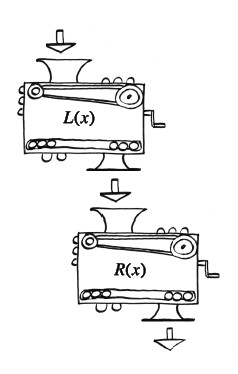Home > A2C > Chapter 6 > Lesson 6.1.2 > Problem6-33

6-33.

Lacey and Richens each have their own personal function machines. Lacey's, $L\left(x\right)$, squares the input and then subtracts one. Richen's function, $R\left(x\right)$, adds $2$ to the input and then multiplies the result by three.

1. Write the equations that represent $L\left(x\right)$ and $R\left(x\right)$.

$L\left(x\right) = x^{2} − 1$

$R\left(x\right) = 3\left(x + 2\right)$

2. Lacey and Richens decide to connect their two machines, so that Lacey's output becomes Richens' input. Eventually, what is the output if $3$ is the initial input?

$30$

3. What if the order of the machines was changed? Would it change the output? Justify your answer.

Try computing the result for $x = 3$ if the order is reversed.